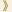HomeShaw Laureates2011Mathematical SciencesEssay

After Newton's introduction of calculus and in particular differential equations to describe the motion of the planets, classical physics and geometry developed with more complex phenomena naturally being formulated in terms of partial differential equations.  The Einstein equations in general relativity and the Ricci Flow equation in Riemannian geometry are two celebrated geometric partial differential equations.  The first describes the geometry of four dimensional spacetime and it relates gravitation to curvature.  The second gives an evolution of Riemannian geometries in which the flow at a given time is dictated by the curvature of the space at that time.  Both of these equations are very elegant in their formulation.  They are nonlinear partial differential equations in several unknown quantities which in turn depend on several variables.  While they are of quite different characteristics in terms of the classification of such equations, they share the feature that they are notoriously difficult to study rigorously (even on a computer).  Central to the understanding of the solutions, is whether they form singularities or not, and if so what is their nature.  In the spacetime setting, examples of singularities are black holes and more generally gravitational collapse.  In the Ricci Flow, should singularities arise in the course of the evolution, then for certain applications they need to be resolved.  Christodoulou, in the case of Einstein's equations, and Hamilton in the case of the Ricci Flow, have made many of the fundamental breakthroughs in the theory of these geometric equations and especially in understanding their singularities. Their works have spectacular applications both to mathematics and to physics.

Demetrios Christodoulou
Christodoulou works in mathematical physics and in particular the differential equations that describe classical physical phenomena.  His continued and profound study of the global behavior of solutions to the Einstein equations has been instrumental in shaping our present day understanding of the critical features of these solutions.  In particular, his striking recent proof of the dynamical development of  trapped surfaces in the setting of Einstein's equations in a vacuum shows that black holes can be formed solely by the interaction of gravitational waves. Earlier, he had made a breakthrough study of the reduced spherically symmetric Einstein equations, showing that naked singularities can occur for these but that they are rare and unstable.  This work resolved the much debated problem of "weak cosmic censorship" for these symmetrically reduced cases. His joint work with S. Klainerman establishing the long sought after nonlinear stability of Minkowski spacetime, is by now one of the classical theorems in the theory.  In other very novel works, Christodoulou has given the first detailed treatment of the delicate problem of the formation of singularities, called shocks, in the equations for fluid flow in three dimensions.  Christodoulou's work combines a deep understanding of the underlying physics with brilliant mathematical technique.  This has allowed him to resolve central problems that have resisted progress for generations.

Richard Hamilton
Hamilton's work is in geometric analysis.  He has provided the theory and one of the most powerful tools to study the shapes (in other words the topology, where we allow deforming but no tearing) of low dimensional spaces.  He introduced the Ricci Flow on the space geometries on a given shape as a means of deforming a given geometry into a regular one.  An early success of his theory was his proof that any positively curved three dimensional space is the ordinary three dimensional sphere.  This opened the door for him to attack the much more general problem of classifying all three dimensional shapes.  This classification problem for two dimensional spaces has been understood for over 100 years.  Thanks to the work of Thurston, one had, by 1980, a strong expectation of what the corresponding classification in three dimensions should be.  It is formulated in Thurston's Geometrization Conjecture, which itself is a far reaching extension of the well-known Poincare Conjecture.  During the past three decades, Hamilton developed a host of original techniques to study the long time behavior of the geometry under his Ricci Flow.  Among these is his technique of surgery allowing for a continuation of the flow should singularities form.  In particular, his methods allowed him to classify four dimensional positively curved spaces.  He has also pursued the application of his techniques in the study of related geometric flows, such as the curve shortening flow and the Gauss and the mean curvature flows.  His ideas and techniques have been used by others to resolve a number of long standing problems in topology and geometry.  In particular, Hamilton's program in three dimensions was completed in brilliant work of Perelman leading to a complete solution of the Conjectures of Poincare and Thurston.  This classification of three dimensional shapes constitutes one of the finest achievements in mathematics.

Mathematical Sciences Selection Committee
The Shaw Prize

28 September 2011, Hong Kong